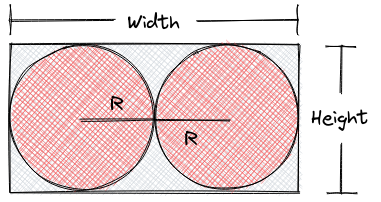# Circles in a Rectangledipto98Intra LU Junior Programmi...
Limits 1s, 512 MB

Two circles are placed inside a rectangle. They do not intersect with each other but they are touching each other. They both have the same radius. Each side of the rectangle are also touched by the circles.Now you are given the radius of the circles. You have to find the area of the green region.

For this problem assume that $π$ (pi) is 3.1416.

## Input

The input starts with an integer $T$ ($T < 11$), denoting the number of test cases.

Each test case contains a floating point number $r$ ($0 < r ≤ 1000$), denoting the radius of the circles. You can assume that $r$ has at most four digits after the decimal point.

## Output

For each test case print the case number as "Case X:" and the area of the green region with two digits after the decimal point.

## Sample

InputOutput
3
30
40.082
85.8

Case 1: 1545.12
Case 2: 2758.15
Case 3: 12638.46GeometryuDebug

### Submit